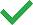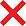# Summary statistics - Cloud

## Talend Cloud Data Management Platform Studio User Guide

Version
Cloud
Language
English (United States)
Product
Talend Cloud
Module
Talend Management Console
Talend Studio
Content
Design and Development

They perform statistical analyses on numeric data, including the computation of location measures such as the median and the average, the computation of statistical dispersions such as the inter quartile range and the range.

• Mean: computes the average of the records.
• Median: computes the value separating the higher half of a sample, a population, or a probability distribution from the lower half.
• Inter quartile range: computes the difference between the third and first quartiles.
• Lower quartile (First quartile): computes the first quartile of data, that is the lowest 25% of data.
• Upper quartile (Third quartile): computes the third quartile of data, that is the highest 25% of data.
• Range: computes the difference between the maximum and minimum values.

When using the summary statistics indicators to profile a DB2 database, analysis results could be slightly different between Java and SQL engines. This is because indicators are computed differently depending on the database type, and also Talend uses special functions when working with Java.

The following table shows the indicators that you can select in any database:

Data type Number Text Date Others
Analysis engine type Java SQL Java SQL Java SQL Java SQL
MeanMedianInter Quartile RangeUpper QuartileRangeMinimumMaximum